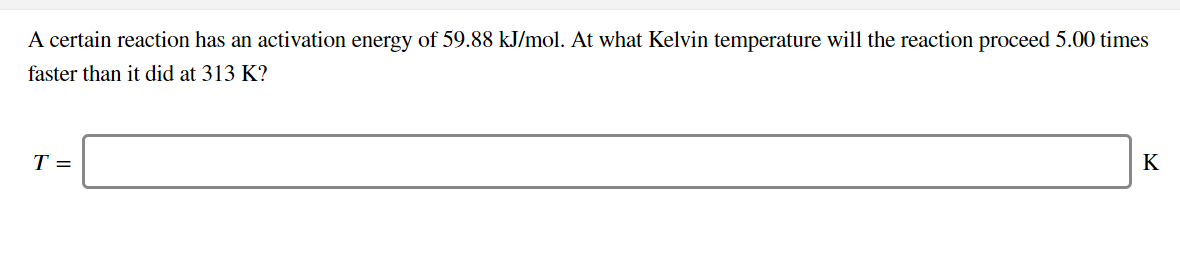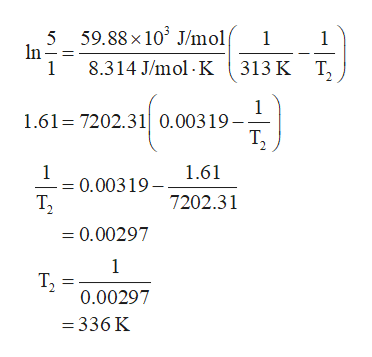# A certain reaction has an activation energy of 59.88 kJ/mol. At what Kelvin temperature will the reaction proceed 5.00 timesfaster than it did at 313 K?T =K

Question
3 viewshelp_outlineImage TranscriptioncloseA certain reaction has an activation energy of 59.88 kJ/mol. At what Kelvin temperature will the reaction proceed 5.00 times faster than it did at 313 K? T = K fullscreen
check_circle

Step 1

The expression for the ratio of two rate constants at the given temperature is given by Arrhenius equation labelled in the equation (1); k1 is the initial rate constant at temperature T1, k2 is the final rate constant at temperature T2, Ea is the activation energy and R is the universal gas constant.

Step 2

The activation energy of the given reaction is 59.88 kJ/mol which is equal to 59.88 × 103 J/mol because 1 kJ/mol ...help_outlineImage Transcriptionclose5 59.88 x 10' J/mol In 1 1 1 8.314 J/mol K313 K T 1 1.61 7202.310.00319- Т, 0.00319-1.61 T2 1 7202.31 =0.00297 1 T2 0.00297 = 336 K fullscreen

### Want to see the full answer?

See Solution

#### Want to see this answer and more?

Solutions are written by subject experts who are available 24/7. Questions are typically answered within 1 hour.*

See Solution
*Response times may vary by subject and question.
Tagged in

### Chemical Kinetics